# Test: Properties Of Inverse Trigonometry

## 15 Questions MCQ Test Mathematics (Maths) Class 12 | Test: Properties Of Inverse Trigonometry

Description
This mock test of Test: Properties Of Inverse Trigonometry for JEE helps you for every JEE entrance exam. This contains 15 Multiple Choice Questions for JEE Test: Properties Of Inverse Trigonometry (mcq) to study with solutions a complete question bank. The solved questions answers in this Test: Properties Of Inverse Trigonometry quiz give you a good mix of easy questions and tough questions. JEE students definitely take this Test: Properties Of Inverse Trigonometry exercise for a better result in the exam. You can find other Test: Properties Of Inverse Trigonometry extra questions, long questions & short questions for JEE on EduRev as well by searching above.
QUESTION: 1

### Evaluate sin(3 sin–1 0.4)​

Solution:

3sin^-1(x) = sin^-1(3x - 4x^3) when -1/2<=x<=1/2
Definitely 0.4 comes in this range of x and so

3sin^-1(0.4) = sin^-1[3*0.4 - 4*0.4^3]
3sin^-1(0.4) = sin^-1[1.2 - 4*0.064]
3sin^-1(0.4) = sin^-1[1.2 - 0.256]
3sin^-1(0.4) = sin^-1[0.944]

Finally , sin(3sin^-1(0.4)) = sin{sin^-1(0.944)} = 0.944

QUESTION: 2

### Evaluate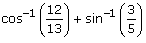Solution:

Explanation : cos-1(12/13) + sin-1 (3/5)

⇒ sin-1 5/13 + sin-1 3/5

Using the formula,  sin-1x +  sin-1y = sin-1( x√1-y² + y√1-x² )

⇒ sin-1 ( 5/13√1-9/25 + 3√1-25/169)

⇒ sin-1 ( 5/13 × 4/5 + 3/5 × 12/13)

⇒ sin-1 (30 + 36 / 65)

⇒ sin-1 (56/ 65)

QUESTION: 3

### If ab + bc + ca = 0, then find 1/a2-bc + 1/b2 – ca + 1/c2- ab

Solution:

1/(a² - bc)   + 1/(b² - ca)   + 1/(c² - ab)
ab + bc + ca = 0
=> bc = -a(b + c)
=> -bc = a(b+c)
a² - bc = a² + a(b + c) = a(a + b + c)
Similarly b² - ca = b(b + a + c) = b(a + b + c)
c² - ab  = c(a + b + c)
= 1/a(a + b + c)    + 1/b(a + b + c)   + 1/c(a + b + c)
=  bc/abc(a + b + c)  + ac/abc(a + b + c)  + ab/abc(a + b + c)
= (bc + ac + ab)/abc(a + b + c)
= (ab + bc + ac)/abc(a + b + c)
= 0/abc(a + b + c)
= 0

QUESTION: 4

If |x| < 1 then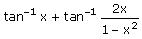Solution: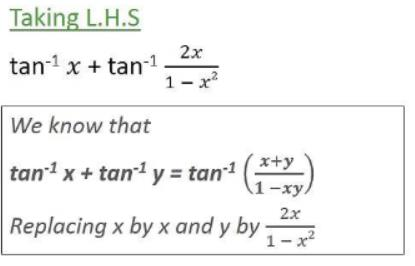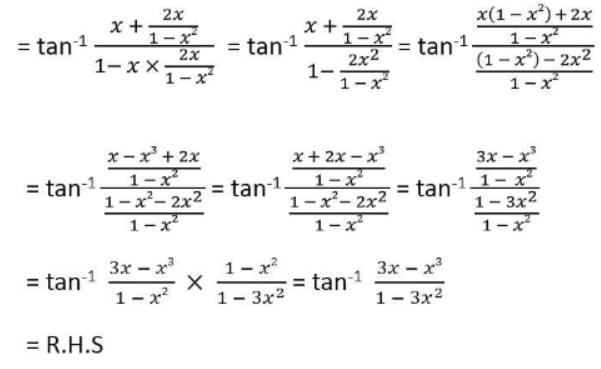QUESTION: 5

sec-1x + cosec-1x =​

Solution:

sec-1x + cosec-1x =​ π/2 ; x belongs to [ -1 , 1 ]

QUESTION: 6

sin 200+ cos 2000 is

Solution:

Because both sin 200and cos 2000 lies in 3rd quadrant. In 3rd quadrant values of both sine and cosine functions are negative.

QUESTION: 7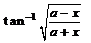is equal to

Solution:

Let y = tan−1[(a−x)/(a+x)]1/2
put x = a cos θ
Now, y = tan−1[(a − a cos θ)/(a + a cos θ)]1/2
⇒ y = tan−1((1 − cos θ)/(1 + cos θ))1/2
⇒ y = tan−1[[(1−cos θ)(1−cos θ)]/(1+cos θ)(1−cos θ)]1/2
⇒ y = tan−1[(1−cos θ)2/1 − cos2θ]1/2
⇒ y = tan−1[(1 − cos θ)/sin θ]
⇒ y = tan−1[2 sin2(θ/2)/2 sin(θ/2) . cos(θ/2)]
⇒ y = tan−1[tan(θ/2)]
⇒ y = θ/2
⇒ y = 1/2 cos−1(x/a)

QUESTION: 8

The principal value of cos–1 (cos 5) is

Solution:
QUESTION: 9

The simplest form of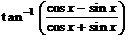Solution:

(cosx−sinx)/(cosx+sinx) = (1−tanx)/(1+tanx)
tan(A−B) = (tanA−tanB)/(1 + tanAtanB)
= tan(π/4−x)
putting this value in question.
tan−1tan(π/4−x)
π/4−x.

QUESTION: 10

The simplest form of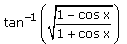for x > 0 is …​

Solution:

tan-1(1-cosx/1+cosx)½
= tan-1{(2sin2 x/2) / (2cos2 x/2)}½
= tan-1{(2sin2 x/2) / (2cos2 x/2)}
= tan-1(tan x/2)
= x/2

QUESTION: 11

Evaluate: sin (2 sin–10.6)​

Solution:

Let, sin-1(0.6) = A…………(1)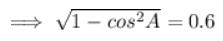( Since, sin² A + cos² A = 1 ⇒ sin² A = 1 - cos² A ⇒ sin A = √(1-cos² A) )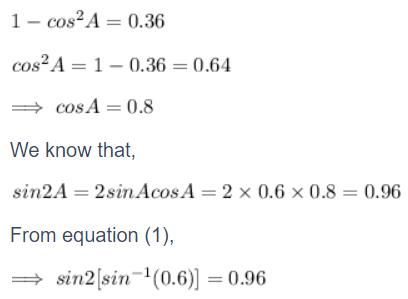QUESTION: 12

The principal value of cos–1 (cos 5) is

Solution:
QUESTION: 13

Value of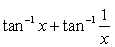is​

Solution:

Assume x>0, then
= tan−1 x + tan−1 (1/x)
= tan−1 x + tan−1(1/tan tan−1 x)
= tan−1 x + tan−1 cot tan−1 x
= tan−1 x + tan−1 tan(π/2−tan−1x)
= tan−1 x + π/2 − tan^−1 x   (∵ for x>0, π2−tan−1 x∈(0,π/2))
= π/2.

QUESTION: 14

Value of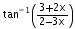Solution:

tan-1[(3+2x)/(2-3x)]
=> tan-1[2(3/2 + x)/2(1-3/2x)]
=> tan-1[(3/2 + x)/(1-3/2x)]
=> tan-1(3/2) + tan-1(x)

QUESTION: 15

Evaluate :cos (tan–1 x)

Solution:

tan−1 x = θ , so that  x=tanθ . We need to determine  cosθ .
sec2θ = 1 + tan2θ = 1 + x2
∴s ecθ = ±√(1+x2
Then, cos(tan−1x) = cosθ=1/secθ = ±1/√(1+x2)